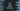# Dart: math library Point class## Point class in dart, its properties and methods :

Dart math library, dart:math provides different mathematical functions and constants. One of its utility class is the Point class. This class is useful to represent two dimensional positions. It provides a couple of different functions and properties used in two dimensional plan coordinate calculations.

In this post, I will show you how to use this class and its utility functions and properties.

### How to use this class :

This class is in the dart:math library. So, we need to import this library to use it :

``import 'dart:math';``

### Constructor of this class :

This class is used to represent one coordinate point on a two dimensional plan. It has one constructor that takes the x and y values for that point :

``Point(T x, T y)``

Where, T must extend num.

### Properties of this class :

Two main properties of this class are magnitude and hashCode.

hashCode returns the hash code of this object. This is a integer value.

magnitude returns the distance between (0,0) and this point. It is also called Euclidean distance.

### Methods of this class :

It provides the following methods :

#### distanceTo(Point p2)-> double :

Returns the distance of the current Point and the point p2.

#### squaredDistanceTo(Point p2)-> T :

It returns the squared distance of the current point and the other point p2.

#### toString() -> string :

This method returns the string representation of this Point object.

### Example :

Let’s consider the below example :

``````main(List<string> args) {
var point1 = Point(10,10);
var point2 = Point(20,20);
print("magnitude of point1 : \${point1.magnitude}");
print("Distance between point1 and point2 : \${point1.distanceTo(point2)}");
print("Squared Distance between point1 and point2 : \${point1.squaredDistanceTo(point2)}");
}``````

It will print :

``````magnitude of point1 : 14.142135623730951
Distance between point1 and point2 : 14.142135623730951
Squared Distance between point1 and point2 : 200``````